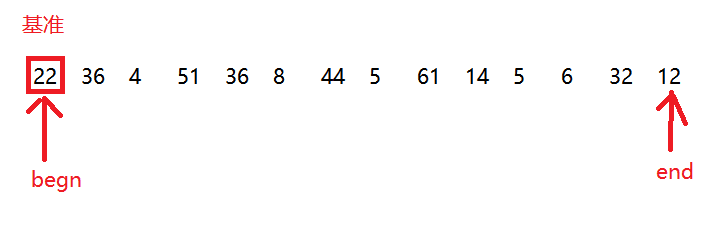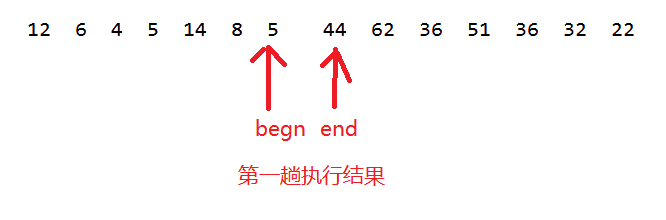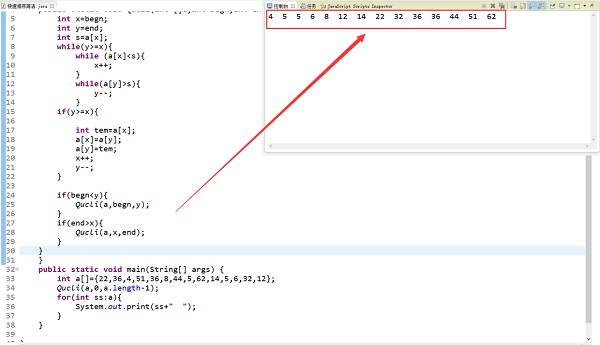一、什么叫快速排序？

通过一趟排序将要排序的数据分割成独立的两部分，其中一部分的所有数据都比另外一部分的所有数据都要小，然后再按此方法对这两部分数据分别进行快速排序，整个排序过程可以递归进行，以此达到整个数据变成有序序列。

二、排序步骤：

对下列数组进行排序：（22,36,4,51,36,8,44,5,62,14,5,6,32,12）

1.）找出一个元素作为基准（理论上可以随便找一个）定义两个指针begn和end.

Java代码
1. int x=begn;
2. int y=end;
3. int s=a[begn];2.）排序实现：

1. 以 22 位基准 ;

2. 在begn位置开始往上找大于等于22的数字；

3.在end处开始往下找小于等于22的数字；

4.找到后交换位置

Java代码
1. while (a[x]<s){
2.     x++;
3. }
4. while(a[y]>s){
5.     y--;
6. }
7.  if(y>=x){
8.     int tem=a[x];
9.     a[x]=a[y];
10.     a[y]=tem;
11.     x++;
12.     y--;
13. }

第一趟执行结果：3.）最后重复以上操作调用递归算法：

Java代码
1. if(begn<y){
2.     Qucli(a,begn,y);
3. }
4. if(end>x){
5.     Qucli(a,x,end);
6. }

三、完整程序：

Java代码
1. public class QuickSort {
2.   //快速排序
3.     public static void Qucli(int []a,int begn,int end){
4.         int x=begn;
5.         int y=end;
6.         int s=a[begn];
7.         while(y>=x){
8.             while (a[x]<s){
9.                 x++;
10.             }
11.             while(a[y]>s){
12.                 y--;
13.             }
14.         if(y>=x){
15.
16.             int tem=a[x];
17.             a[x]=a[y];
18.             a[y]=tem;
19.             x++;
20.             y--;
21.         }
22.
23.         if(begn<y){
24.             Qucli(a,begn,y);
25.         }
26.         if(end>x){
27.             Qucli(a,x,end);
28.         }
29.     }
30.     }
31.     public static void main(String[] args) {
32.         int a[]={22,36,4,51,36,8,44,5,62,14,5,6,32,12};
33.         Qucli(a,0,a.length-1);
34.         for(int ss:a){
35.             System.out.print(ss+"  ");
36.         }
37.     }
38.
39. }

结果展示：2017年1月6日